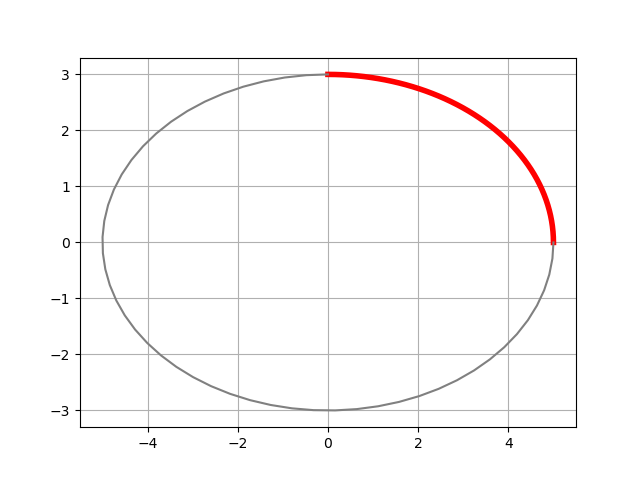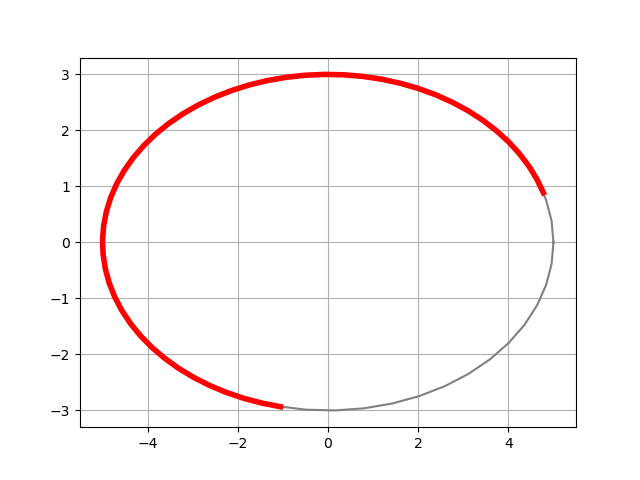# How to calculate length of an elliptic arc

This post will show how to find the length of piece of an ellipse and explain what elliptic integrals have to do with ellipses.

Assume we have an ellipse centered at the origin with semi-major axis a and semi-minor axis b. So a > b > 0, the longest diameter of the ellipse is 2a and the shortest is 2b. The ellipse can be parameterized by## Special case: quarter of an ellipse

Let’s first find the perimeter of a 1/4 of the ellipse.This is given by the integralThe change of variables u = π/2 – t turns the integral intobecause E, the “elliptic integral of the second kind,” is defined byTherefore the perimeter of the entire ellipse is 4aE(1 – b²/a²). Let’s definefor the rest of the post. Incidentally, m = e² where e is the eccentricity of the ellipse.

## General case

Now let’s find the length of an arc where t ranges from 0 to T and T is not necessarily π/2.The derivation is similar to that above.where E, now with two arguments, is the “incomplete elliptic integral of the second kind” defined byIt is “incomplete” because we haven’t completed the integral by integrating all the way up to π/2.

To find the length of a general arc whose parameterization runs from t = T0 to t = T1 we find the length from 0 out to T1 and subtract the length from 0 out to T0 which gives usThe elliptic integrals are so named because they came out of the problem we’re looking at in this post. The integrals cannot be computed in elementary terms, so we introduce new functions that are defined by the integrals. I expand this idea in this post.

NB: We are specifying arcs by the range of our parameterization parameter t, not by the angle from the center of the ellipse. If our ellipse were a circle the two notions would be the same, but in general they are not. The central angle θ and the parameter T are related via## Python code

Let’s calculate the length of an arc two ways: using our formula and using numerical integration. Note that the Python implementation of the (complete) elliptic integral is ellipe and the implementation of the incomplete elliptic integral is ellipeinc. The “e” at the end of ellipe distinguishes this elliptic integral, commonly denoted by E, from other kinds of elliptic integrals.


from numpy import pi, sin, cos
from scipy.special import ellipe, ellipeinc

def arclength(T0, T1, a, b):
m = 1 - (b/a)**2
t1 = ellipeinc(T1 - 0.5*pi, m)
t0 = ellipeinc(T0 - 0.5*pi, m)
return a*(t1 - t0)

def numerical_length(T0, T1, a, b):
f = lambda t: (a**2*sin(t)**2 + b**2*cos(t)**2)**0.5